Author: Oscar Cronquist Article last updated on September 19, 2022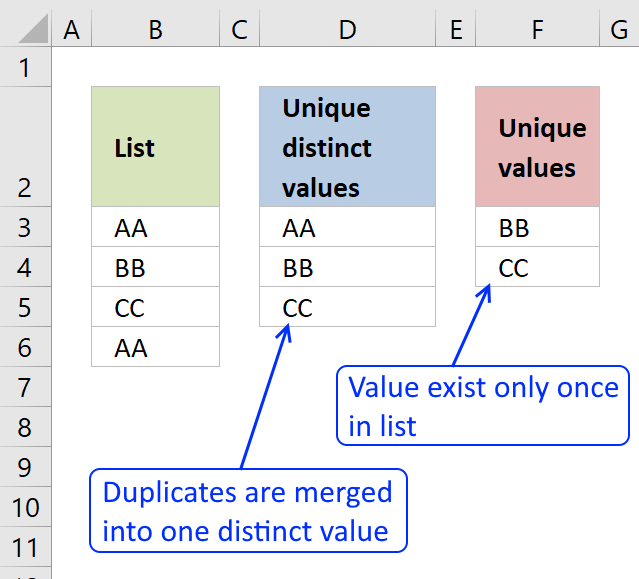First, let me explain the difference between unique values and unique distinct values, it is important you know the difference so you can find the information you are looking for on this web page.

The picture above shows a list of values in column B, note value AA has a duplicate. Unique distinct values are all cell values but duplicate values are merged into one distinct value. In other words, duplicates are removed only one instance of each value is left in the list.

Column F contains unique values from column B, meaning values that exist only once in column B. Value AA is not in column F because it has a duplicate, in other words, AA is not unique in column B. To filter duplicates, read this post: Extract a list of duplicates from a column

### What you will learn in this article

• The difference between unique distinct values and unique values.
• How to decide which Excel feature to use.
• How to use a formula that extracts unique distinct values.
• How to copy the values returned by the formula.
• How the formula works and the functions being used.
• How to filter unique distinct values considering lowercase and uppercase letters.
• How to filter unique distinct values using the Advanced Filter.
• How to highlight unique distinct values using Conditional Formatting.
• How to build a User defined Function that filters unique distinct values sorted from A to Z.
• Where to put the VBA code.
• How to enter and use the User defined Function.
• How to filter unique values using a formula.
• How to highlight unique values using Conditional Formatting.

### What is possible with formulas?

You have quite a few options to choose from if you are looking for a way to create a unique distinct list in your workbook, all demonstrated in this post or on this website. Not only an exceptionally small regular formula, if you want to use that, but also awesome built-in features in Excel that makes your work so much easier.

Formulas are very versatile, they allow you to build solutions for very specific tasks like filtering unique distinct values from two separate columns or three. If your list contains blanks then this article is for you: Extract a unique distinct list and remove blanks

Perhaps you want to do a wildcard lookup and return unique distinct values or simply return unique distinct values based on a condition.

I have also written articles that explains how to create a unique distinct list sorted alphabetically, sum or frequency.

There is also a formula for extracting unique distinct values located in a multi-column cell range, it is a somewhat more complicated array formula, however, there is a custom function as well, if you prefer that.

### What is the easiest way to filter unique distinct values?I would choose the advanced filter if you are not looking for a formula. It lets you quickly filter a unique distinct list.

If you know that you will be extracting unique distinct values from time to time, like in a dashboard or an interactive worksheet, I recommend using a formula and an Excel defined table. You won't need to repeat the same steps over and over compared to the advanced filter and that will save you time and repetitive work.

However working with a large data set may slow down the formula calculations considerably depending on your computer hardware, so perhaps the User Defined Function [UDF] is a better choice or even better a pivot table, if you have huge amounts of data to work with.

The Excel Pivot table is lightning fast even with huge data tables but it does have a little learning curve and it requires a few steps to set it up but in my opinion, it is totally worth learning how to use pivot tables. You will be surprised how easy it is to start working with Excel Pivot tables.

Conditional Formatting allows you to format cells determined by a built-in rule or a formula you construct. In this post, you will find a Conditional Formatting formula that highlights unique and unique distinct values. Did you know that you can easily sort highlighted values on top? Check out conditional formatting.

I have made an add-in that lets you extract unique, unique distinct and duplicate values and records from multiple worksheets. This allows you to easily bring together data from multiple sources in your workbook.

There is also a useful array formula in this article that extracts a case-sensitive unique distinct list, this is a special case which the built-in Excel tools can't accomplish.

## 1. Create a list of unique distinct values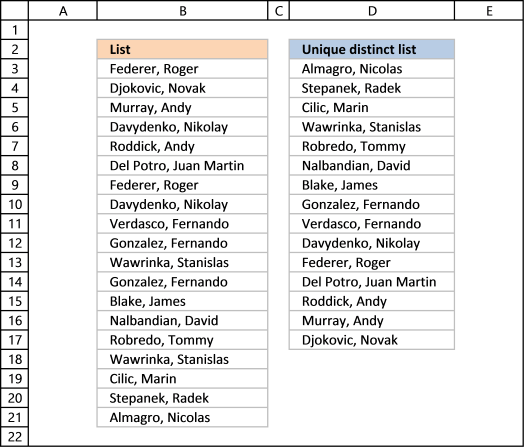Column B contains names, some cells have duplicate values.  A formula in column D extracts a unique distinct list from column B.

Update: 2017-08-15!

This formula is even smaller than the array formula and you are not required to enter this as an array formula. The following formula is for older Excel versions than Excel 365 subscribers.

Formula in cell D3:

=LOOKUP(2,1/(COUNTIF(\$D\$2:D2,\$B\$3:\$B\$21)=0),\$B\$3:\$B\$21)

I will explain how this formula works in the video below and in section 1.3 also below.

Update: 2020-05-28!

Microsoft Excel released new functions for Excel 365 subscribers in January 2020. One of those new functions is the UNIQUE function, it allows you to easily extract a unique distinct list using only one function.

Formula in cell D3:

=UNIQUE(B3:B21)

This formula is entered as a regular formula, however, it is a dynamic array formula. Microsoft Excel introduced dynamic array formulas in January 2020 as well.

Dynamic array formulas expand to cells below automatically if more than one value is returned from the formula. Microsoft Excel calls this behavior spilling. You can find more example of the UNIQUE function here.

I will describe a formula for older Excel versions below.

### 1.1 Video

This video demonstrates how to use the formula:

Subscribe to Get Digital Help on Youtube:

### 1.2 Copy unique distinct values

To copy unique distinct values to another location you must make sure you copy the values and not the formula:

1. Select list
2. Copy list, shortcut keys: CTRL + C or press this button:
3. Press with right mouse button on on destination cell and press with left mouse button on the black arrow next to "Paste Special..."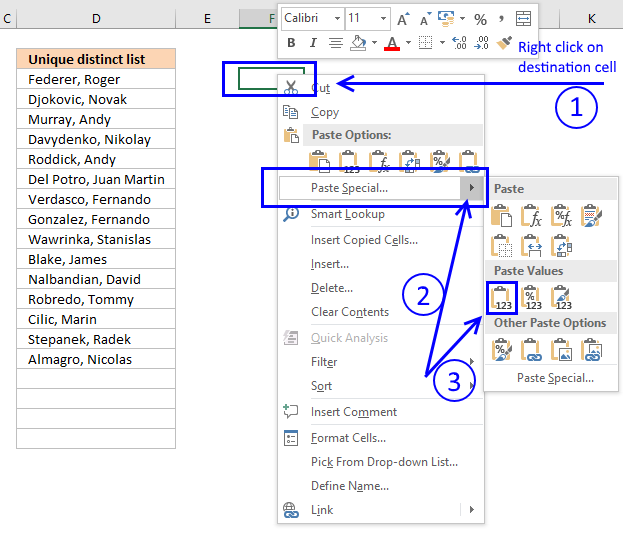4. Then press with left mouse button on "Paste Values" button.

### 1.3 Explaining formula in cell D3

#### Step 1 - Count previous values above the current cell

The COUNTIF function allows you to count values based on a condition. With the help from an expanding cell reference, the formula knows which of the values that have been extracted.

In cell D3 no values have been extracted so it compares the value in the cell above current cell, this happens to be the Header value. Make sure you don't have a value in the list that matches the header value, it won't be extracted.

COUNTIF(\$D\$2:D2,\$B\$3:\$B\$21) is entered in column F, displayed in the picture below.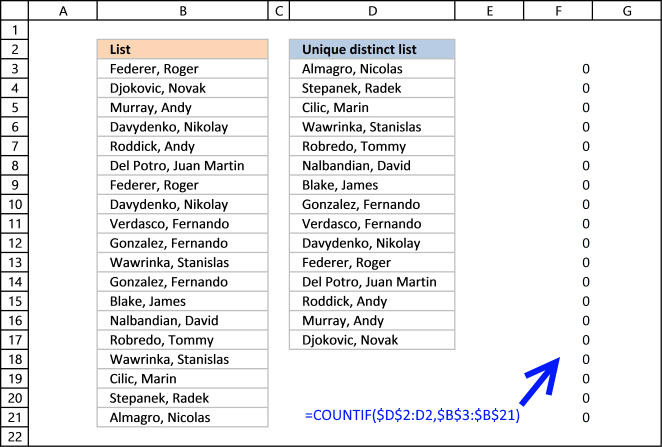The value in cell D2 is not found in any instance in cell range B3:B21, all values in the array are 0 (zero). Note that the array has the same size as the list in column B, 19 values.

#### Step 2 - Compare array with 0 (zero)

To identify values that have not been shown the formula compares the array with 0 (zero) and the result are boolean values (TRUE or FALSE) for each value in the array.

COUNTIF(\$D\$2:D2,\$B\$3:\$B\$21) = 0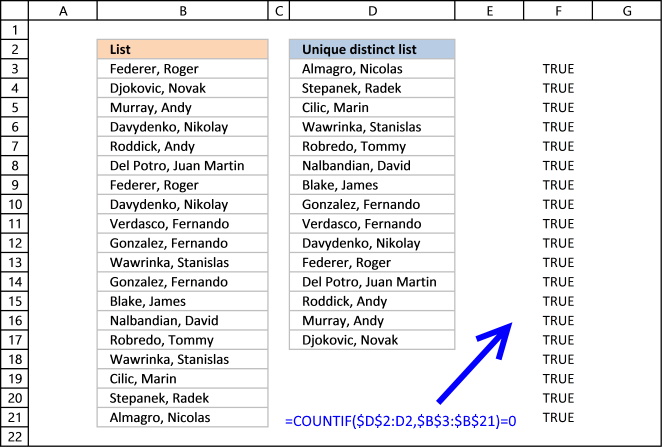The array contains 19 boolean values, all TRUE.

#### Step 3 - Divide 1 with array

The boolean value TRUE is equal to 1 and FALSE is equal to 0. If a value in the array is TRUE the result will be 1 because 1/TRUE equals 1.

If a value in the array is FALSE the result will be #DIV0! because 1/FALSE is 1/0 and you can't divide a number with zero. Excel returns an error.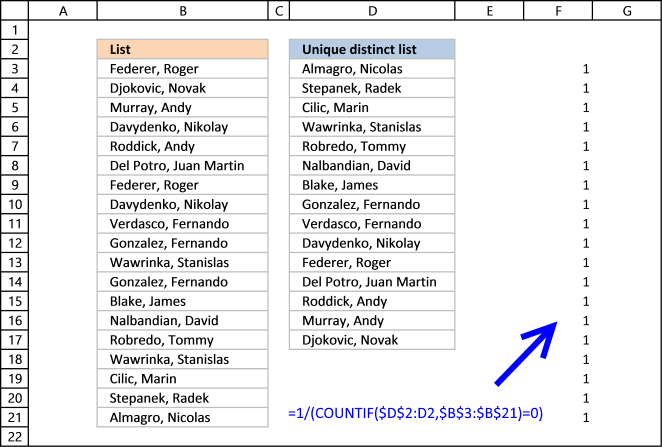The good thing about the LOOKUP function is that it ignores errors, see next step.

#### Step 4 - LOOKUP value

The LOOKUP function is designed to work with sorted cell ranges or arrays, you get weird results if they are not sorted. Be careful using the LOOKUP function.

However, in this case, the values in the array are either 1 or #DIV0!. Surprisingly it ignores errors, the only thing it can find then is a value that is 1.

The first argument in the LOOKUP function is 2 so the function finds the last largest value that is equal to 2 or smaller.

LOOKUP(2,1/(COUNTIF(\$D\$2:D2,\$B\$3:\$B\$21)=0),\$B\$3:\$B\$21)

becomes

LOOKUP(2,{1;1;1;1;1;1;1;1;1; 1;1;1;1;1;1;1;1;1;1},\$B\$3:\$B\$21) and matches the last value in the array. LOOKUP function then returns the corresponding value in cell range \$B\$3:\$B\$21 which is Almagro, Nicolas

### Get the Excel fileExtract-a-unique-distinct-list-in-excelv5.xlsx

## 2. Extract a unique distinct list (case sensitive)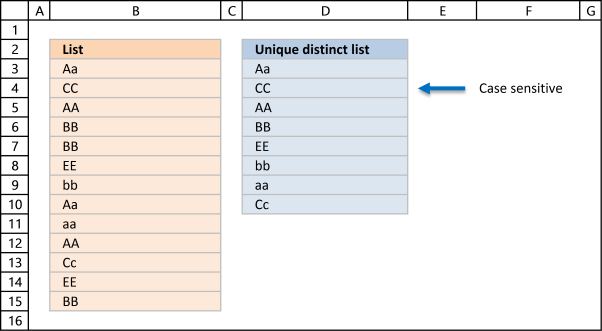The following array formula lists unique distinct values from a list also considering upper and lower letters. For example, the value "Aa" is not equal to "AA".

Array formula in cell D3:

=INDEX(\$B\$3:\$B\$15, MATCH(0, FREQUENCY(IF(EXACT(\$B\$3:\$B\$15, TRANSPOSE(\$D\$2:D2)), MATCH(ROW(\$B\$3:\$B\$15), ROW(\$B\$3:\$B\$15)), ""), MATCH(ROW(\$B\$3:\$B\$15), ROW(\$B\$3:\$B\$15))), 0))

Excel 365 subscribers can use this regular somewhat shorter formula in cell D3 than the formula below:

=LET(z, B3:B15, x, SEQUENCE(z), INDEX(z, MATCH(0, FREQUENCY(IF(EXACT(z, TRANSPOSE(\$D\$2:D2)), x, ""), x), 0))

The formula above contains two new formulas: LET function and the SEQUENCE function.

This article explains the formula: Extract a case sensitive unique list from a column - Excel 365

### 2.1 Video

This video demonstrates how to build a formula that extracts a case-sensitive unique distinct list:

Subscribe to Get Digital Help on Youtube:

This post shows you how to extract a case sensitive unique list from a column:

Recommended articles

How to extract a case sensitive unique list from a column
This article demonstrates a formula that extracts unique values from a column also considering upper and lower characters (case sensitive). […]

How to enter an array formula

### 2.2 Explaining the array formula in cell C3

Step 1 - Transpose previous values

TRANSPOSE(\$D\$2:D2)

becomes

TRANSPOSE({"Unique distinct list (case sensitive)";"Aa"})

and returns

{"Unique distinct list (case sensitive)","Aa"}

Note that the ; (semicolon) changes to a , (comma)

Recommended articles

How to use the TRANSPOSE function
The TRANSPOSE function allows you to convert a vertical range to a horizontal range, or vice versa. A vertical range […]

Step 2 - Check if two text strings are exactly the same, also case sensitive

EXACT(\$B\$3:\$B\$15, TRANSPOSE(\$D\$2:D2))

becomes

EXACT(\$B\$3:\$B\$15, TRANSPOSE({"Unique distinct list (case sensitive)","Aa"})

becomes

EXACT(\$B\$3:\$B\$15, TRANSPOSE({"Unique distinct list (case sensitive)","Aa"})

becomes

EXACT({"Aa"; "CC"; "AA"; "BB"; "BB"; "EE"; "bb"; "Aa"; "aa"}, TRANSPOSE({"Unique distinct list (case sensitive)","Aa"})

and returns

{FALSE, TRUE; FALSE, FALSE; FALSE, FALSE; FALSE, FALSE; FALSE, FALSE; FALSE, FALSE; FALSE, FALSE; FALSE, TRUE; FALSE, FALSE}

Step 3 - Return relative position in array if TRUE

IF(EXACT(\$B\$3:\$B\$15, TRANSPOSE(\$C\$1:C1)), MATCH(ROW(\$B\$3:\$B\$15), ROW(\$B\$3:\$B\$15))

becomes

IF({FALSE, TRUE; FALSE, FALSE; FALSE, FALSE; FALSE, FALSE; FALSE, FALSE; FALSE, FALSE; FALSE, FALSE; FALSE, TRUE; FALSE, FALSE}, MATCH(ROW(\$A\$1:\$A\$9), ROW(\$A\$1:\$A\$9)))

becomes

IF({FALSE, TRUE; FALSE, FALSE; FALSE, FALSE; FALSE, FALSE; FALSE, FALSE; FALSE, FALSE; FALSE, FALSE; FALSE, TRUE; FALSE, FALSE}, {1;2;3;4;5;6;7;8;9})

and returns

{FALSE,1; FALSE,FALSE; FALSE,FALSE; FALSE,FALSE; FALSE,FALSE; FALSE,FALSE; FALSE,FALSE; FALSE,8; FALSE,FALSE}

Recommended article:

Recommended articles

How to use the COUNTIF function
Counts the number of cells that meet a specific condition.

Step 4 - Calculate how often values exist in an array

FREQUENCY(IF(EXACT(\$B\$3:\$B\$15, TRANSPOSE(\$C\$1:C1)), MATCH(ROW(\$B\$3:\$B\$15), ROW(\$B\$3:\$B\$15)), ""), MATCH(ROW(\$B\$3:\$B\$15), ROW(\$B\$3:\$B\$15)))

becomes

FREQUENCY({FALSE,1; FALSE,FALSE; FALSE,FALSE; FALSE,FALSE; FALSE,FALSE; FALSE,FALSE; FALSE,FALSE; FALSE,8; FALSE,FALSE},MATCH(ROW(\$B\$3:\$B\$15),ROW(\$B\$3:\$B\$15)))

becomes

FREQUENCY({FALSE,1; FALSE,FALSE; FALSE,FALSE; FALSE,FALSE; FALSE,FALSE; FALSE,FALSE; FALSE,FALSE; FALSE,8; FALSE,FALSE},{1;2;3;4;5;6;7;8;9})

and returns

{1;0;0;0;0;0;0;1;0;0} Aa is found in position 1 and 8 in cell range \$B\$3:\$B\$15

Recommended articles

How to use the FREQUENCY function
Returns how many times values exist in a given range. Note, this function returns an array of values.

Step 5 - Find first empty value (0) in array

MATCH(0, FREQUENCY(IF(EXACT(\$B\$3:\$B\$15, TRANSPOSE(\$C\$1:C1)), MATCH(ROW(\$B\$3:\$B\$15), ROW(\$B\$3:\$B\$15)), ""), MATCH(ROW(\$B\$3:\$B\$15), ROW(\$B\$3:\$B\$15))), 0)

becomes

MATCH(0, {1;0;0;0;0;0;0;1;0;0}, 0)

and returns 2.

Recommended articles

How to use the MATCH function
Identify the position of a value in an array.

Step 6 - Return value from position 2

INDEX(\$B\$3:\$B\$15, MATCH(0, FREQUENCY(IF(EXACT(\$B\$3:\$B\$15, TRANSPOSE(\$C\$1:C1)), MATCH(ROW(\$B\$3:\$B\$15), ROW(\$B\$3:\$B\$15)), ""), MATCH(ROW(\$B\$3:\$B\$15), ROW(\$B\$3:\$B\$15))), 0))

becomes

INDEX(\$B\$3:\$B\$15, 2)

and returns "CC" in cell C3.

Recommended articles

How to use the INDEX function
Gets a value in a specific cell range based on a row and column number.

### Get the Excel fileExtract-a-unique-distinct-list-in-excel4.xlsx

Recommended articles

## 3. Extract unique distinct values [Advanced Filter]

First a little reminder, unique distinct values are all cell values but duplicate values are merged into one distinct value.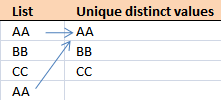### 3.1 Video

The following video shows you how to filter unique distinct values using Advanced Filter:

Subscribe to Get Digital Help on Youtube:

### 3.2 Instructions - Copy unique distinct values to another location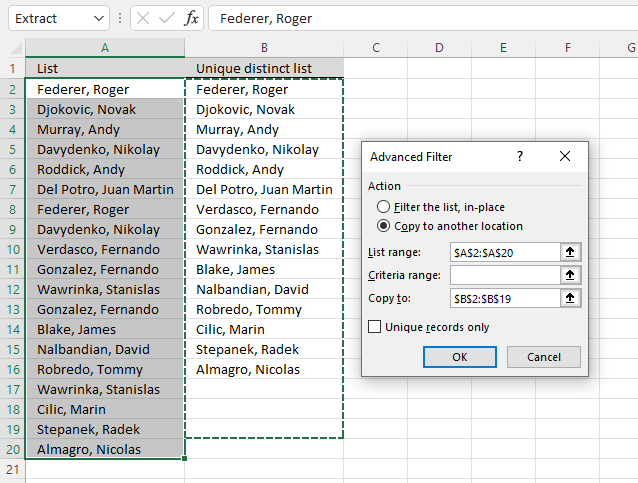This section describes how to extract unique distinct values using the built-in feature "Advanced Filter".

1. Go to tab "Data" on the ribbon.
2. Press the "Advanced Filter" button on the ribbon.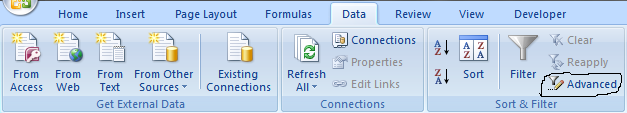3. Press button "Copy to another location".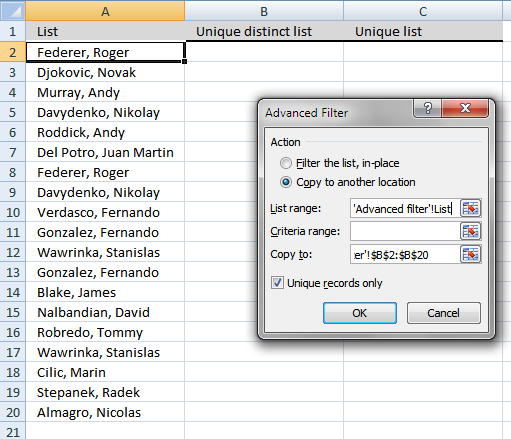4. Press "List range:" and select range to filter unique distinct values.
5. Press "Copy to: and select a range.
6. Press "Unique records only" button to select it.
7. Press with left mouse button on "OK" button to apply settings and start extracting.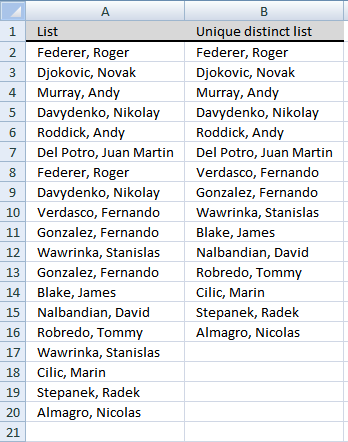### 3.3 Instructions - Filter unique distinct values, in place

If you choose to filter unique distinct values in-place, press with left mouse button on the first option button in the dialog box.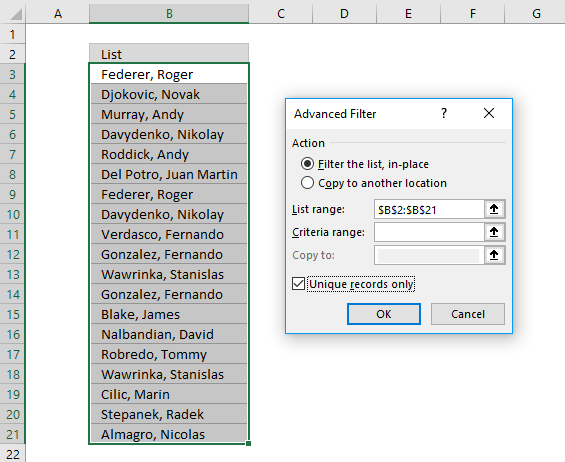You can then select unique distinct values and paste to another location, duplicate values are hidden and are ignored when you copy cell range B3:21 and paste to a new location, very useful.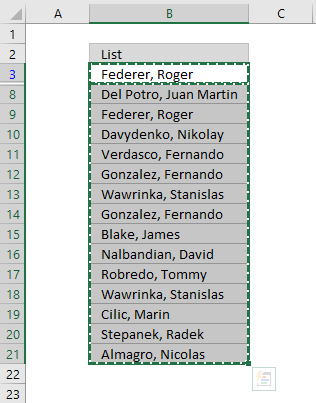The picture below shows you the selected distinct values after I cleared the Advanced Filter, duplicate values are not selected because they were hidden.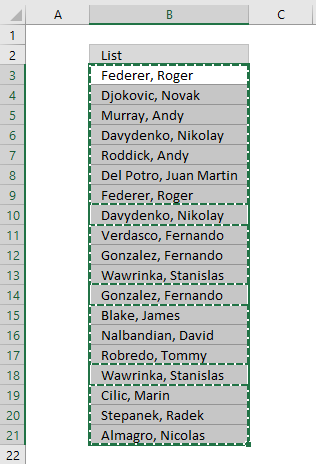Recommended articles

An Advanced Filter is not the only powerful built-in feature in Excel, I highly recommend that you learn pivot tables. Perhaps the most powerful tool but also the least known:

Recommended articles

How to use Pivot Tables – Excel’s most powerful feature and also least known
A pivot table allows you to examine data more efficiently, it can summarize large amounts of data very quickly and is very easy to use.

The Excel defined table is also extremely useful, it allows you to quickly sort, filter and manipulate data. Learn that and much more:

Recommended articles

How to use Excel Tables
An Excel table allows you to easily sort, filter and sum values in a data set where values are related.

## 4. Highlight unique distinct values [Conditional Formatting]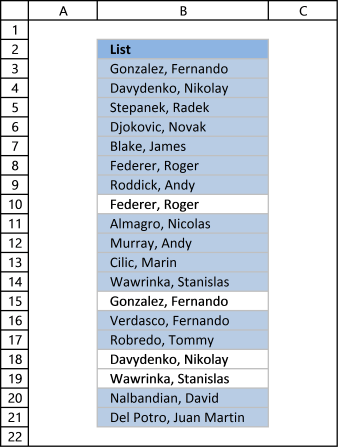This section demonstrates how to highlight unique distinct values using Excel's built-in feature "Conditional Formatting".

The image shows you unique distinct values highlighted using Conditional Formatting.

### 4.1 Video

This video demonstrates how to highlight unique distinct values:

Subscribe to Get Digital Help on Youtube:

### How to highlight unique distinct values

1. Select cell range B3:B21.
2. Go to tab "Home" on the ribbon.
3. Press on "Conditional Formatting" button.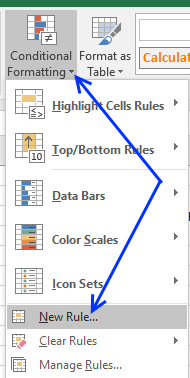4. Press on "New Rule...".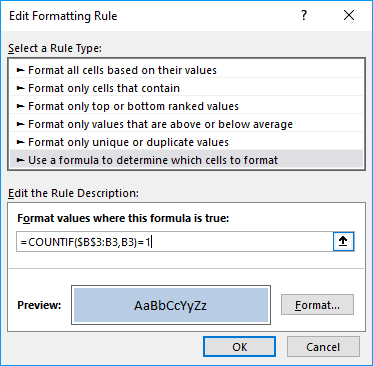5. Press on "Use a formula to determine which cells to format:".
6. Type this formula: =COUNTIF(\$B\$3:B3,B3)=1
7. Press on "Format..." button.
8. Pick a color.
9. Press OK button.
10. Press OK button again.

### 4.2 Explaining Conditional Formatting formula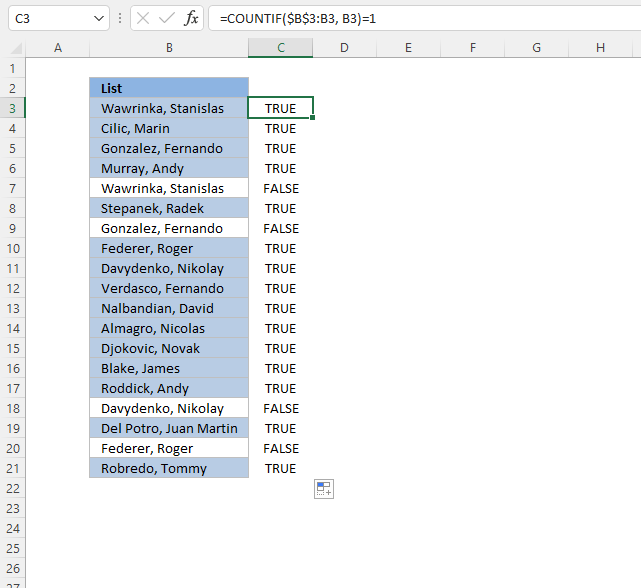A CF formula works somewhat differently than a regular formula, however, they may be harder to spot.

It is possible that you can't even see if a cell range has CF applied to it or not, if no cells are highlighted.

I recommend that you copy the CF formula and enter it to an adjacent column to better show how they work.

#### Step 1 - COUNTIF function

The COUNTIF function has two arguments, the first argument is the cell range you want to count a specific value in. The second argument is the value you want to count.

COUNTIF(rangecriteria)

#### Step 2 - COUNTIF arguments

The first argument uses both relative and absolute cell references, \$B\$3:B3. The absolute part has dollar signs \$B\$3 meaning it does not change when the Conditional Formatting formula is applied to the next cell.

The relative part B3 does change when the Conditional Formatting formula is applied to the next cell.

COUNTIF(\$B\$3:B3, B3)

#### Step 3 - Demonstrate calculations in cells B3 and B4

In cell B3 the function is COUNTIF(\$B\$3:B3,B3)

and in cell B4: COUNTIF(\$B\$3:B4,B4) and so on.

This technique using growing cell references lets you highlight the first instance of a value but not duplicate values.

#### Step 4 - Compare output to 1

How do we know if the value is a unique distinct value? Compare COUNTIF(\$B\$3:B3,B3) to 1 and it will return TRUE or FALSE, like this:

COUNTIF(\$B\$3:B3,B3)=1

The equal sign is a logical operator that returns TRUE or FALSE. Note, the comparison is not case sensitive. The output is a boolean value TRUE or FALSE.

COUNTIF(\$B\$3:B3,B3)=1

becomes

1=1

and returns boolean value TRUE. Cell B3 is highlighted.

If COUNTIF(\$B\$3:B3,B3) returns a number larger than 1 meaning there is at least a duplicate value in the cell range specified in the first argument. That prevents the Conditional Formatting formula from highlighting the cell.

### 4.3 Sort Conditional formatted cells at the top

Tip! Press with right mouse button on on a highlighted cell, press with left mouse button on Sort and then on "Put Selected Cell Color On top" to arrange unique distinct values at the very top of your list.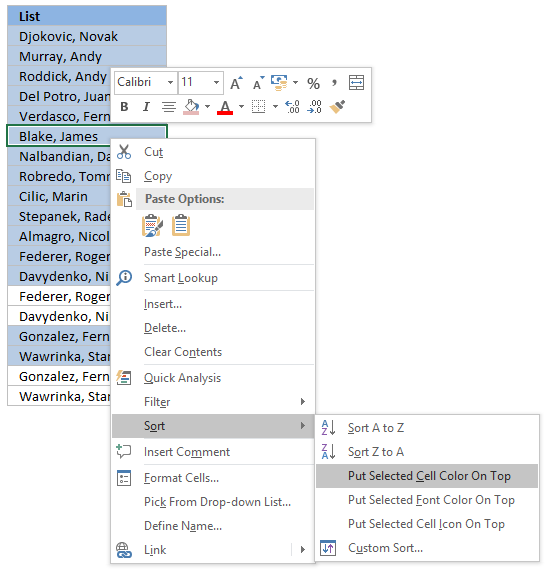The picture below shows you all unique distinct values sorted together.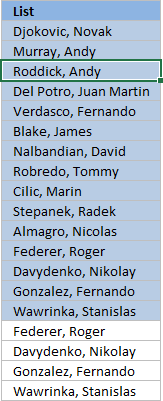## 5. Hide duplicate values [Conditional Formatting]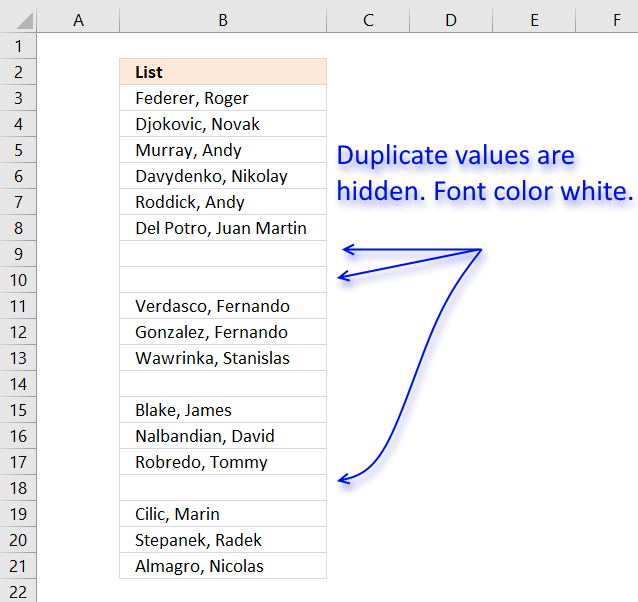The image above demonstrates Conditional Formatting applied to a list of values, it changes the font color to white for duplicate values making them invisible or they appear hidden.

Keep in mind that the text is still there so if you copy the range and paste the values to a new range the hidden values are visible again. I recommend that you sort the visible values at the top in order to copy them correctly, instructions below.

Conditional Formatting formula:

=COUNTIF(\$B\$3, B3)>1

How to apply conditional formatting formula to cell range B3:B21

1. Select cell range B3:B21.
2. Go to tab "Home" on the ribbon.
3. Press with left mouse button on the "Conditional Formatting" button.
4. Press with left mouse button on "New Rule..."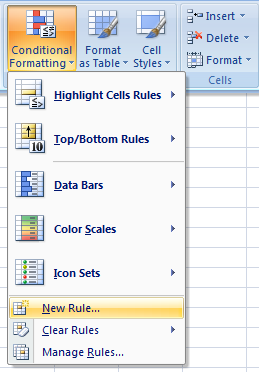5. Press with left mouse button on "Use a formula to determine which cells to format:".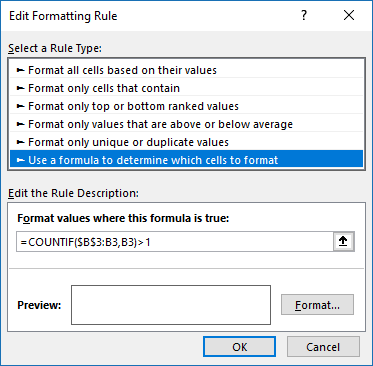6. Type the Conditional Formatting formula in "Format values where this is true:".
7. Press with left mouse button on "Format..." button.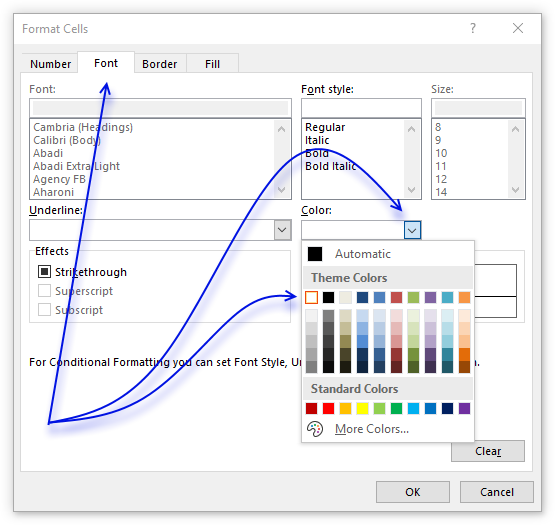8. Go to tab "Font" on the menu, see image above.
9. Press with left mouse button on color drop-down list.
10. Pick white.
11. Press with left mouse button on OK button.
12. Press with left mouse button on OK button.
13. Press with left mouse button on OK button.

## 6. How to sort unique distinct values at the top of the list

1. Press with right mouse button on on one of the visible values in the list.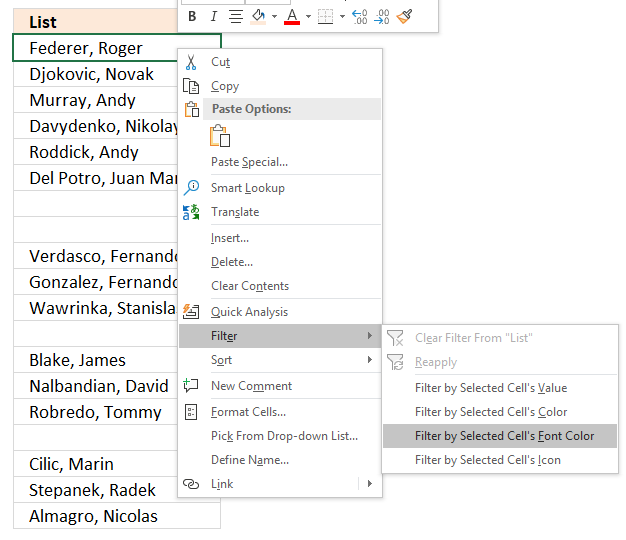2. Press with left mouse button on "Filter"
3. Press with left mouse button on "Filter by Selected Cell's Font color.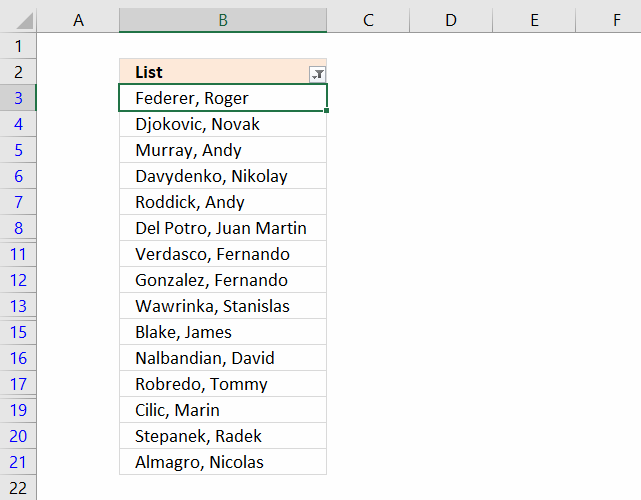## 7. Extract unique distinct sorted values from a cell range [UDF]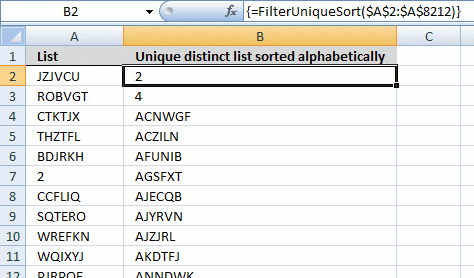This UDF lets you create and sort a unique distinct list. First you need to copy the VBA code to your workbook, instructions below. Second, select a cell range. Third, type FilterUniqueSort(cell_ref) in the formula bar. Last, enter formula as an array formula, instructions below.

There is also a workbook for you to get.

Array formula in cell B2:B8212:

=FilterUniqueSort(\$A\$2:\$A\$8212)

### 7.1 Video

This video explains how to implement and use the User Defined Function

Subscribe to Get Digital Help on Youtube:

### 7.2 How to create an array formula

1. Type B2:B8212 in name box
2. Type above array formula in formula bar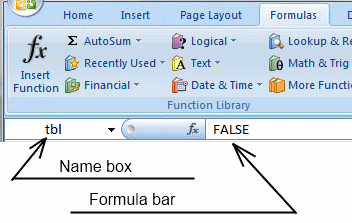3. Press and hold Ctrl + Shift
4. Press Enter once
5. Release all keys

Recommended articles

A beginners guide to Excel array formulas
Array formulas allows you to do advanced calculations not possible with regular formulas.

### 7.3 VBA code

I am using the selection sort function to sort values. You can read more about the function here:

Using a Visual Basic Macro to Sort Arrays in Microsoft Excel

```'Name User Defined Function and define paremeter
Function FilterUniqueSort(rng As Range)

'Dimension variables and declare data types
Dim ucoll As New Collection, Value As Variant, temp() As Variant
Dim iRows As Single, i As Single

'Redimension array variable
ReDim temp(0)

'Enable error handling
On Error Resume Next

'Iterate through each value in range
For Each Value In rng

'Check if number of characters in value is greater than 0 (zero), if true add value to collection ucoll
If Len(Value) > 0 Then ucoll.Add Value, CStr(Value)

'Continue with next value
Next Value

'Disable error handling
On Error GoTo 0

'Iterate through each value in collection ucoll
For Each Value In ucoll

'Save value to last container in array variable temp
temp(UBound(temp)) = Value

'Add new container to array variable temp
ReDim Preserve temp(UBound(temp) + 1)

'Next value
Next Value

'Remove last container in array variable temp
ReDim Preserve temp(UBound(temp) - 1)

'Save selected rows on worksheet to variable iRows

'Start User Defined Function SelectionSort with values in array variable temp
SelectionSort temp

'Add blanks to array variable temp to prevent error values on worksheet
For i = UBound(temp) To iRows

ReDim Preserve temp(UBound(temp) + 1)

'Save blank to container
temp(UBound(temp)) = ""

'Continue with next value
Next i

'Transpose values in array variable temp and return those values to worksheet
FilterUniqueSort = Application.Transpose(temp)

End Function
```
```'Name User Defined Function (UDF) and define parameters
Function SelectionSort(TempArray As Variant)
'This UDF sorts values in an array
'https://www.get-digital-help.com/how-to-extract-a-unique-list-and-the-duplicates-in-excel-from-one-column/#7.3

'Dimension variables and declare data types
Dim MaxVal As Variant
Dim MaxIndex As Integer
Dim i As Integer, j As Integer

'Iterate through each value in array variable temp starting from last to first
For i = UBound(TempArray) To 0 Step -1

'Save value to variable MaxVal
MaxVal = TempArray(i)

'Save value stored in variable i to variable MaxIndex
MaxIndex = i

'Iterate through each value in array variable temp
For j = 0 To i

'Check if value in array variable TempArray is larger than value stored in variable MaxVal
'Excel can compare text values as well, this action checks if a text value is before or after another value in a sorted list
If TempArray(j) > MaxVal Then

'If true save value to variable MaxVal
MaxVal = TempArray(j)

'Save position to MaxIndex
MaxIndex = j
End If

'Continue with next value
Next j

'Check if number stored in variable MaxIndex is smaller than number stored in variable i
If MaxIndex < i Then

'Save value in array variable TempArray position i to array variable TempArray container position MaxIndex
TempArray(MaxIndex) = TempArray(i)

'Save value in variable MaxVal to array variable TempArray container position i
TempArray(i) = MaxVal
End If
Next i

End Function
```

### 7.4 Where to copy VBA code?

1. Press Alt + F11 to open VB Editor
2. Press with left mouse button on "Insert" on the menu
3. Press with left mouse button on "Module" to create a module
4. Copy (Ctrl + c) above VBA code and paste (Ctrl +v)  to the code module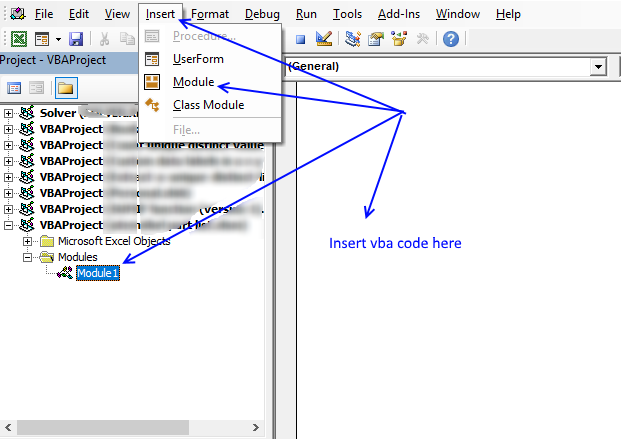### Get the Excel fileExtract-unique-distinct-sorted-values-from-a-cell-range.xls

#### More powerful User Defined Functions

Recommended articles

Filter unique distinct records (case sensitive) [UDF]
The User Defined Function demonstrated above extracts unique distinct records also considering upper and lower case letters. For example, a record […]

Recommended articles

Lookup and return multiple values concatenated into one cell
This article demonstrates how to find a value in a column and concatenate corresponding values on the same row. The […]

Recommended articles

Filter unique distinct words from a cell range [UDF]
This blog post describes how to create a list of unique distinct words from a cell range. Unique distinct words […]

## 8. Filter unique distinct values from multiple sheets add-in

Filter unique distinct values is an add-in for Excel 2007/2010/2013 that lets you extract

• unique distinct values
• duplicate values
• unique distinct records
• duplicate records

from multiple sheets. The Add-In contains 4 user-defined functions.

If a value in one of the ranges changes the function will automatically and instantly update the list.

#### Features

• All user-defined functions remove blank values and blank records.
• No error values when all values are extracted.
• Filter values or records from up to 255 different cell ranges or sheets.

#### 8.1 Watch this video where I demonstrate the Excel Add-In

Subscribe to Get Digital Help on Youtube:

#### What are unique distinct values?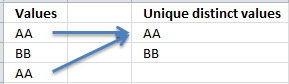#### What are unique distinct records?Purchase Filter Unique Distinct Values From Multiple Sheets Add-in For Excel 2007/2010/2013 - Price \$19 USD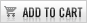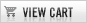### Questions

Is there a money back guarantee?
Sure, you have a unconditional money back guarantee for 14 days.

## 9. Create a list of unique distinct values [Old array Formula]

I recommend using the regular formula above since it is smaller and has an advantage of not being an array formula.Array formula in cell D3:

=INDEX(\$B\$3:\$B\$21, MATCH(0, COUNTIF(\$D\$2:D2, \$B\$3:\$B\$21), 0))

Thanks to Eero, who contributed the original array formula!

The formulas above has an issue with blank cells, it returns a 0 (zero) in your list. This article shows you how to ignore blanks:

Recommended articles

Extract a unique distinct list and ignore blanks
This article demonstrates formulas that extract unique distinct values and ignore blank empty cells. Table of contents Extract a unique […]

### 9.1 How to create an array formula

You don't need to follow these steps if you chose the regular formula.

1. Copy the array formula above (Ctrl + c)
2. Double press with left mouse button on cell B2
3. Paste (Ctrl + v)
4. Press and hold Ctrl + Shift simultaneously
5. Press Enter
6. Release all keys

If you made the above steps correctly the formula now has a beginning and ending curly bracket, like this:
{=INDEX(\$B\$3:\$B\$21, MATCH(0, \$D\$2:D2, \$B\$3:\$B\$21), 0))}

Don't enter these characters yourself, they appear automatically.

Copy cell B2 and paste to cells below as far as needed.

### 9.2 How the array formula in cell B2 works

#### Step 1 - Create an array with the same size as the list

The COUNTIF function calculates the number of cells equal to a condition.

COUNTIF(rangecriteria)

COUNTIF(\$B\$1:B1, \$A\$2:\$A\$20)

becomes

COUNTIF("Unique distinct list",{Federer,Roger; Djokovic,Novak; Murray,Andy; Davydenko,Nikolay; Roddick,Andy; DelPotro,JuanMartin; Federer,Roger; Davydenko,Nikolay; Verdasco,Fernando; Gonzalez,Fernando; Wawrinka,Stanislas; Gonzalez,Fernando; Blake,James; Nalbandian,David; Robredo,Tommy; Wawrinka,Stanislas; Cilic,Marin; Stepanek,Radek; Almagro,Nicolas} )

and returns:

{0;0;0;0;0;0;0;0;0;0;0;0;0;0;0;0;0;0;0}

This means the cell value in \$B\$1:B1 can't be found in any of the cells in cell range \$A\$2:\$A\$20. If it had been found, somewhere in the array the number 1 would exist.

#### Step 2 - Return the position  of an item that matches 0 (zero)

The MATCH function returns the relative position of an item in an array that matches a specified value.

MATCH(lookup_value, lookup_array, [match_type]

MATCH(0, COUNTIF(\$B\$1:B1, \$A\$2:\$A\$20), 0)

becomes

MATCH(0,{0; 0; 0; 0; 0; 0; 0; 0; 0; 0; 0; 0; 0; 0; 0; 0; 0; 0; 0},0)

and returns 1.

#### Step 3 - Return a cell value

The INDEX function returns a value or reference of the cell at the intersection of a particular row and column, in a given range.

INDEX(array, row_num, [column_num])

INDEX(\$B\$3:\$B\$21, 1)

becomes

=INDEX({Federer,Roger; Djokovic,Novak; Murray,Andy; Davydenko,Nikolay; Roddick,Andy; DelPotro,JuanMartin; Federer,Roger; Davydenko,Nikolay; Verdasco,Fernando; Gonzalez,Fernando; Wawrinka,Stanislas; Gonzalez,Fernando; Blake,James; Nalbandian,David; Robredo,Tommy; Wawrinka,Stanislas; Cilic,Marin; Stepanek,Radek; Almagro,Nicolas}, 1)

and returns "Federer, Roger".

Relative and absolute cell references

When you copy the array formula down the countif formula range (\$B\$1:B1) expands. This is created by using relative and absolute references.

The first cell, B2: COUNTIF(\$B\$1:B1,\$A\$2:\$A\$20)

Second cell, B3: COUNTIF(\$B\$1:B2,\$A\$2:\$A\$20)

and so on.

Recommended articles

How to use absolute and relative references
What is a reference in Excel? Excel has an A1 reference style meaning columns are named letters A to XFD […]

## 10. How to filter unique values from a list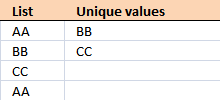Unique values are values existing only once in a list. Example, "AA" exists twice in the list below and is not unique. BB and CC exist only once each and are unique in the list.

Column D in the picture below filters all unique values from column B. Unique values are values that exist only once in column B.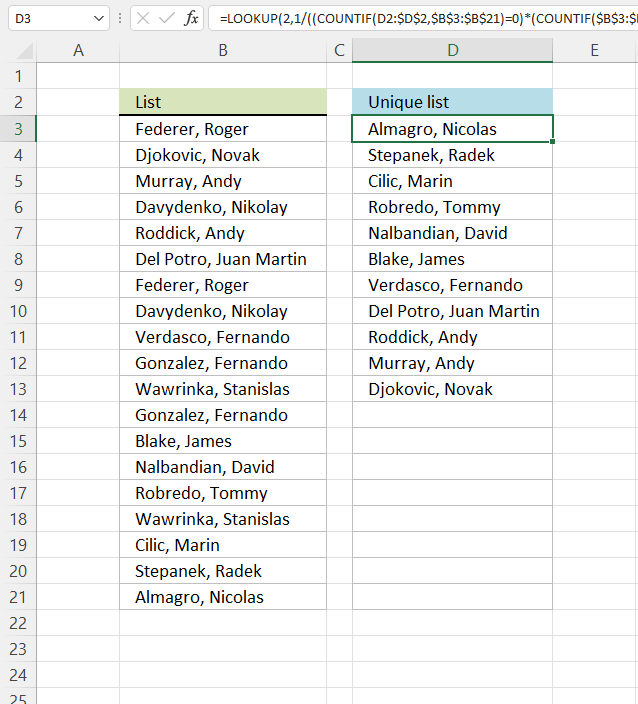Example, Roger, Federer is not in column D because there is more than one value of this name in column A. In other words, the name is not unique in column A. You can find the name twice in the list, in cells A2 and A8.

Update 2017-08-30
This formula is even smaller than the array formula and you are not required to enter this as an array formula.

Regular formula in cell D3:

=LOOKUP(2, 1/((COUNTIF(D2:\$D\$2, \$B\$3:\$B\$21)=0)*(COUNTIF(\$B\$3:\$B\$21, \$B\$3:\$B\$21)=1)), \$B\$3:\$B\$21)

Recommended articles

Update 2020-12-09, the formula below extracts unique values from cell range \$B\$3:\$B\$21:

Regular formula in cell D3:

=UNIQUE(\$B\$3:\$B\$21,,TRUE)

The formula above contains the new UNIQUE function that only Excel 365 subscribers can use. Use the formula above if you have an earlier Excel version.

Recommended articles

Array formula in cell D3:

=INDEX(\$B\$3:\$B\$21, MATCH(0, COUNTIF(D2:\$D\$2, \$B\$3:\$B\$21)+(COUNTIF(\$B\$3:\$B\$21, \$B\$3:\$B\$21)<>1), 0))

How to enter an array formula

### 10.1 Explaining array formula in cell D3

#### Step 1 - Count each value in array and check if it is not equal to one

(COUNTIF(\$A\$2:\$A\$20, \$A\$2:\$A\$20)<>1

becomes

{2;1;1;2;1;1;2;2;1;2;2;2;1;1;1;2;1;1;1}<>1

and returns

{TRUE; FALSE; FALSE; TRUE; FALSE; FALSE; TRUE; TRUE; FALSE; TRUE; TRUE; TRUE; FALSE; FALSE; FALSE; TRUE; FALSE; FALSE; FALSE}

This array tells excel that the first value in the array is not unique and that is true because Roger, Federer is not unique in the list. However the second value is FALSE and that value is unique, etc.

Recommended articles

How to use the COUNTIF function
Counts the number of cells that meet a specific condition.

#### Step 2 - Keep track of previous values

C1:\$C\$1 is a dynamic cell reference, it changes as the formula is copied to cells below. You can read more about absolute and relative cell references here:

Recommended articles

How to use absolute and relative references
What is a reference in Excel? Excel has an A1 reference style meaning columns are named letters A to XFD […]

COUNTIF(C1:\$C\$1, \$A\$2:\$A\$20)

becomes

COUNTIF("Unique list", {"Federer, Roger "; "Djokovic, Novak "; "Murray, Andy "; "Davydenko, Nikolay "; "Roddick, Andy "; "Del Potro, Juan Martin "; "Federer, Roger "; "Davydenko, Nikolay "; "Verdasco, Fernando "; "Gonzalez, Fernando "; "Wawrinka, Stanislas "; "Gonzalez, Fernando "; "Blake, James "; "Nalbandian, David "; "Robredo, Tommy "; "Wawrinka, Stanislas "; "Cilic, Marin "; "Stepanek, Radek "; "Almagro, Nicolas "})

and returns

{0; 0; 0; 0; 0; 0; 0; 0; 0; 0; 0; 0; 0; 0; 0; 0; 0; 0; 0}

A zero (0) means that no values have yet been displayed and that is true in cell C2. However when excel calculates the value in cell C3, cell C2 shows "Djokovic, Novak" and the array becomes {0; 1; 0; 0; 0; 0; 0; 0; 0; 0; 0; 0; 0; 0; 0; 0; 0; 0; 0}. The second value in the array contains 1. This tells excel that value has already been shown.

#### Step 3 - Add arrays

COUNTIF(C1:\$C\$1, \$A\$2:\$A\$20)+(COUNTIF(\$A\$2:\$A\$20, \$A\$2:\$A\$20)<>1

becomes

{TRUE; FALSE; FALSE; TRUE; FALSE; FALSE; TRUE; TRUE; FALSE; TRUE; TRUE; TRUE; FALSE; FALSE; FALSE; TRUE; FALSE; FALSE; FALSE} + {0; 0; 0; 0; 0; 0; 0; 0; 0; 0; 0; 0; 0; 0; 0; 0; 0; 0; 0}

and returns

{1;0;0;1;0;0;1;1;0;1;1;1;0;0;0;1;0;0;0}

TRUE is 1 and FALSE is zero. So True + 0 equals 1 and False + 1 equals 1.

#### Step 4 - Find first zero value in array

A zero in the array indicates {1;0;0;1;0;0;1;1;0;1;1;1;0;0;0;1;0;0;0} that the corresponding value is unique and has not yet been displayed in the list.

MATCH(0, COUNTIF(C1:\$C\$1, \$A\$2:\$A\$20)+(COUNTIF(\$A\$2:\$A\$20, \$A\$2:\$A\$20)<>1), 0)

becomes

MATCH(0, {1;0;0;1;0;0;1;1;0;1;1;1;0;0;0;1;0;0;0}, 0)

and returns 2.

Recommended articles

How to use the MATCH function
Identify the position of a value in an array.

#### Step 5 - Return corresponding value

INDEX(\$A\$2:\$A\$20, MATCH(0, COUNTIF(C1:\$C\$1, \$A\$2:\$A\$20)+(COUNTIF(\$A\$2:\$A\$20, \$A\$2:\$A\$20)<>1), 0))

becomes

INDEX(\$A\$2:\$A\$20, 2)

and returns "Djokovic, Novak" in cell C2.

Recommended articles

How to use the INDEX function
Gets a value in a specific cell range based on a row and column number.

### Get the Excel fileExtract-a-unique-distinct-list-in-excelv7.xlsx

To extract duplicates, see this post:

Recommended articles

Extract a list of duplicates from a column
The array formula in cell C2 extracts duplicate values from column A. Only one duplicate of each value is displayed […]

## 11. Highlight unique values [Conditional Formatting]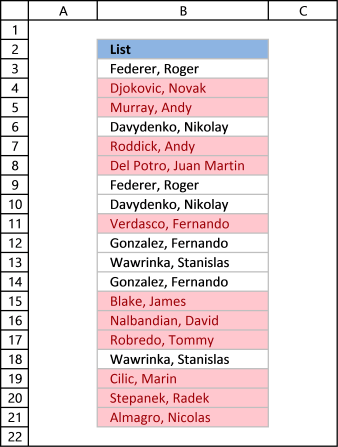This example demonstrates how to highlight cells with a color of your choice if it contains a unique value.

### 11.1 Video

The following video shows you how to color unique values using conditional formatting. Remember, it highlights only unique values, in other words, values that exist only once in the list.

Subscribe to Get Digital Help on Youtube:

Instructions

1. Go to tab "Home" on the ribbon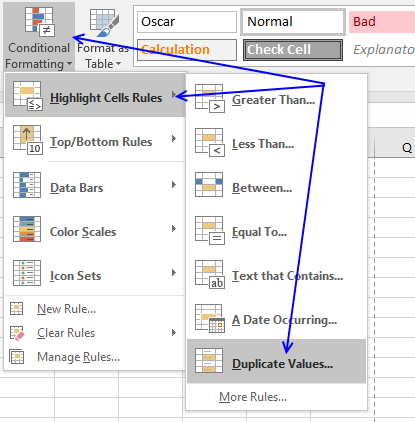2. Press with left mouse button on "Conditional Formatting" button
3. Hover over "Highlight Cell Rules"
4. Press with mouse on "Duplicate Values..."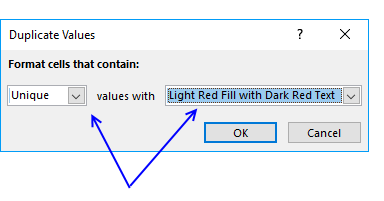5. Press with mouse on the leftmost drop-down list and change it to "Unique"
6. Pick a formatting if you like
7. Press with left mouse button on OK buttonThe picture above shows you, for example, that the first name in the list has a duplicate so that name is not highlighted in any cell.

### 11.2 Sort unique values at the top

Tip! Did you know that you can put highlighted values to the top

1. Press with right mouse button on on a highlighted cell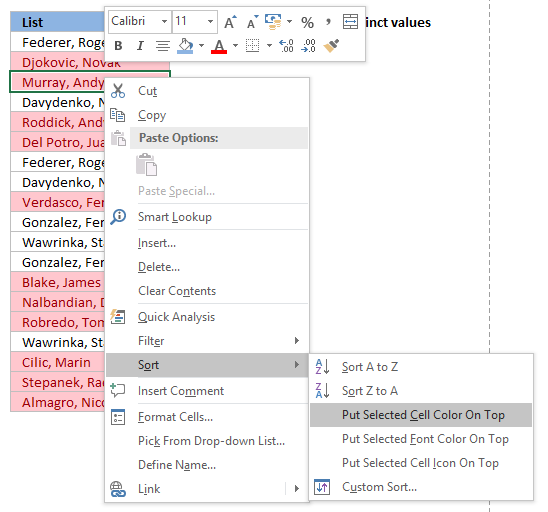2. Press with mouse on "Sort"
3. Press with mouse on "Put Selected Cell Color On Top"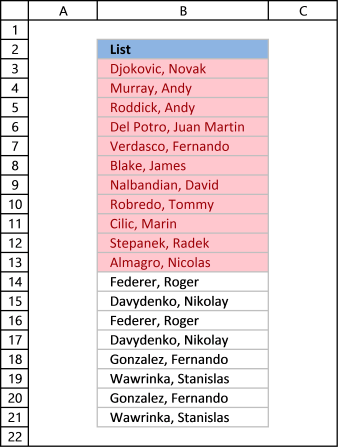## 12. Useful tips

### 12.1 Excel tables

An Excel Table is a great feature and is very cleverly designed. It is constructed to automatically expand if you add more data which is incredibly helpful. You don't need to do anything, not adjusting cell references which is time consuming and prone to errors.

Structured references are cell references to an excel defined table. They let you easily see what the data contains as long as you give it good descriptive column header names.

I recommend you use excel defined tables instead of named ranges or dynamic named ranges as long as you are working with more than one value.

Here is how to convert a list to an excel defined table: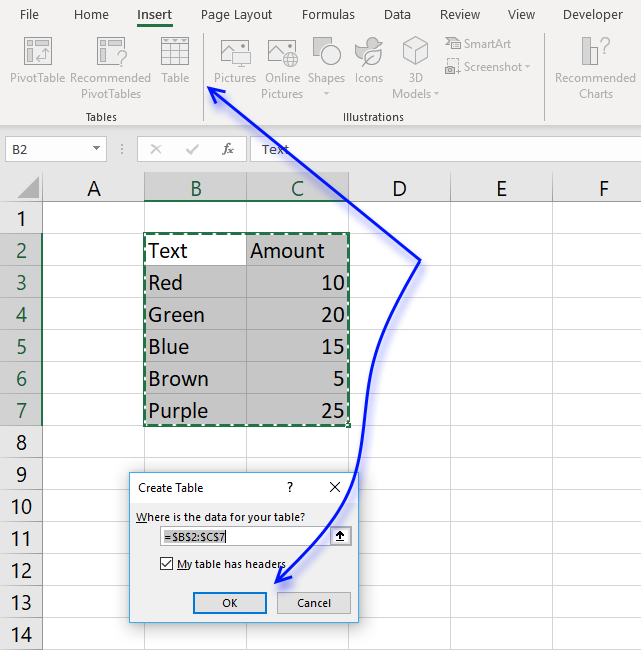1. Select a cell in your list
2. Go to tab "Insert" on the ribbon and press with left mouse button on Table button or press Ctrl + T
3. Press with left mouse button on OK button
4. Your excel defined table is created

### 12.2 Named ranges

In excel you can name a cell range, a constant or a formula. You can then use the named range in a formula, making it easier for you to read and understand formulas.

Example

List : A2:A20

Tip! Use dynamic named ranges to automatically adjust cell ranges when new values are added or removed.

#### 12.2.1 How to create a named range

The downside with named ranges is that you need to adjust the range every time you add or delete a value in the list, the named range will then not fit the value list. I recommend using excel defined tables if you know that the list may change in the future.

1. Select cell range B3:B7
2. Type Color in name box
3. Press Enter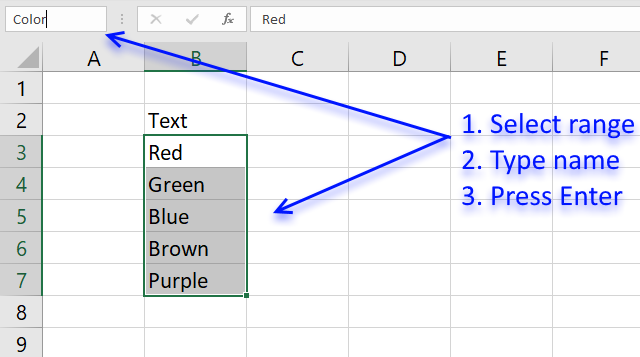Formula example containing name range:

=INDEX(Color,MATCH(0,COUNTIF(\$C\$2:C2,Color),0))

## 13. How to remove errors (Excel 2007)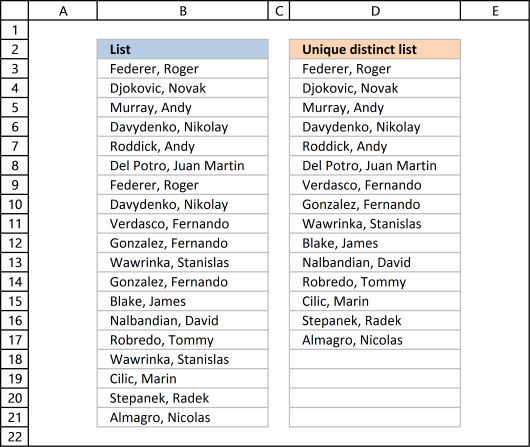Excel 2007 users (and later versions) can remove errors using IFERROR() function.

When the formula runs out of values it returns #N/A errors (Not Available), you can use the IFERROR function to remove the error and return blanks in those cells.

Unfortunately, it comes with a big disadvantage, it also removes other formula errors as well. So use this with great caution. If your source table has errors you won't detect it because the IFERROR function returns a blank cell instead.

Array formula in cell D2:

=IFERROR(LOOKUP(2, 1/(COUNTIF(\$D\$2:D2, \$B\$3:\$B\$21)=0), \$B\$3:\$B\$21), "")

and copy it down as far as necessary.

Recommended articles

## 14. Excel 2003 users can remove errors using isna() function:

=IF(ISNA(INDEX(\$A\$2:\$A\$20, MATCH(0, COUNTIF(\$B\$1:B1, \$A\$2:\$A\$20), 0))), "", INDEX(\$A\$2:\$A\$20, MATCH(0, COUNTIF(\$B\$1:B1, \$A\$2:\$A\$20), 0)))

and copy it down as far as needed.

This formula is an array formula, how to enter an array formula.

## 15. How to ignore blank cells in a range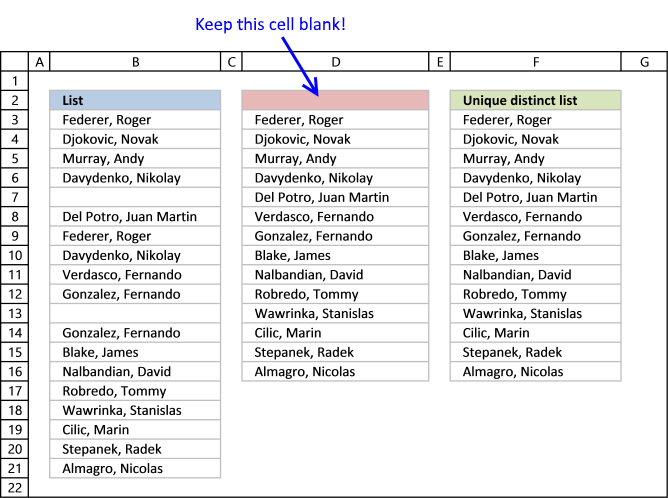Harlan Grove created a formula to count unique distinct values from a list with blanks. I used the same technique here to filter unique distinct values in column D.

If you want a header name you can use the slightly larger formula, displayed in column F below.

Update 2020-12-09, Excel 365 users can use this regular formula:

=UNIQUE(FILTER(\$B\$3:\$B\$21, (\$B\$3:\$B\$21<>"") * (\$B\$3:\$B\$21<>"")))

The formula below is actually 58 character while the new Excel 365 formula above is 61 characters, space characters not included. You can find a formula explanation here: Extract unique distinct values ignoring blanks

Use the formula below if you have an earlier Excel version than Excel 365.

Update 2017-09-01, smaller regular formula in cell D3:

=LOOKUP(2, 1/(COUNTIF(\$D\$2:D2, \$B\$3:\$B\$21&"")=0), \$B\$3:\$B\$21)

Formula in cell F3 if you need a column header name:

=LOOKUP(2, 1/((COUNTIF(\$F\$2:F2, \$B\$3:\$B\$21)+(\$B\$3:\$B\$21=""))=0), \$B\$3:\$B\$21)

#### 15.1 Watch a video where I explain how these two formulas work

Subscribe to Get Digital Help on Youtube:

This article shows you how to fill blank cells with values or formulas

Recommended articles

Learn how to extract non-blank cells in a list using a formula:

Recommended articles

Remove blank cells
In this blog post I will provide two solutions on how to remove blank cells and a solution on how […]